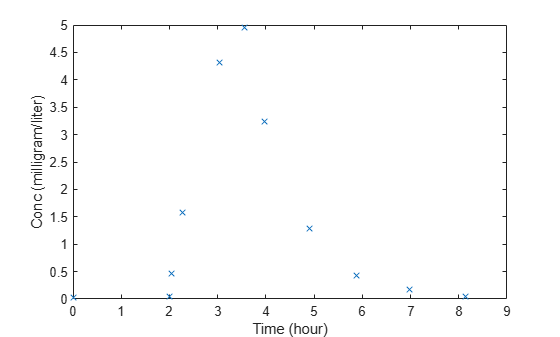# DurationParameterName

Parameter specifying length of time to administer a dose

## Description

`DurationParameterName` is a property of a `RepeatDose` or `ScheduleDose` object.

Specify the name of a parameter object that is scoped to a model. This parameter defines the length of time it takes to administer a dose.

you can parameterize the property by setting it to the name of a model-scoped parameter that is not being modified by a repeated assignment rule, an algebraic rule, or a rate rule. However, the parameter can be modified by an event.

Note

If you set the `DurationParameterName` property of a dose, you must also specify the `Amount` property of the dose, and set the `Rate` property to `0`. This is because the rate is calculated from the amount and duration.

## Characteristics

 Applies to Objects: `RepeatDose`, `ScheduleDose`. Data type Character vector. Data values Name of a model-scoped parameter object. The default value is an empty character vector `''`. Access Read/write.

## Examples

expand all

This example shows how to estimate the time lag before a bolus dose was administered and the duration of the dose using a one-compartment model.

`load lagDurationData.mat`

Plot the data.

```plot(data.Time,data.Conc,'x') xlabel('Time (hour)') ylabel('Conc (milligram/liter)')```Convert to groupedData.

```gData = groupedData(data); gData.Properties.VariableUnits = {'hour','milligram/liter'};```

Create a one-compartment model.

```pkmd = PKModelDesign; pkc1 = addCompartment(pkmd,'Central'); pkc1.DosingType = 'Bolus'; pkc1.EliminationType = 'linear-clearance'; pkc1.HasResponseVariable = true; model = construct(pkmd); configset = getconfigset(model); configset.CompileOptions.UnitConversion = true;```

Add two parameters that represent the time lag and duration of a dose. The lag parameter specifies the time lag before the dose is administered. The duration parameter specifies the length of time it takes to administer a dose.

```lagP = addparameter(model,'lagP'); lagP.ValueUnits = 'hour'; durP = addparameter(model,'durP'); durP.ValueUnits = 'hour';```

Create a dose object. Set the `LagParameterName` and `DurationParameterName` properties of the dose to the names of the lag and duration parameters, respectively. Set the dose amount to 10 milligram which was the amount used to generate the data.

```dose = sbiodose('dose'); dose.TargetName = 'Drug_Central'; dose.StartTime = 0; dose.Amount = 10; dose.AmountUnits = 'milligram'; dose.TimeUnits = 'hour'; dose.LagParameterName = 'lagP'; dose.DurationParameterName = 'durP';```

Map the model species to the corresponding data.

`responseMap = {'Drug_Central = Conc'};`

Specify the lag and duration parameters as parameters to estimate. Log-transform the parameters. Initialize them to 2 and set the upper bound and lower bound.

```paramsToEstimate = {'log(lagP)','log(durP)'}; estimatedParams = estimatedInfo(paramsToEstimate,'InitialValue',2,'Bounds',[1 5]);```

Perform parameter estimation.

`fitResults = sbiofit(model,gData,responseMap,estimatedParams,dose,'fminsearch')`
```fitResults = OptimResults with properties: ExitFlag: 1 Output: [1x1 struct] GroupName: One group Beta: [2x4 table] ParameterEstimates: [2x4 table] J: [11x2 double] COVB: [2x2 double] CovarianceMatrix: [2x2 double] R: [11x1 double] MSE: 0.0024 SSE: 0.0213 Weights: [] LogLikelihood: 18.7511 AIC: -33.5023 BIC: -32.7065 DFE: 9 DependentFiles: {1x2 cell} EstimatedParameterNames: {'lagP' 'durP'} ErrorModelInfo: [1x3 table] EstimationFunction: 'fminsearch' ```

Display the result.

`fitResults.ParameterEstimates`
```ans=2×4 table Name Estimate StandardError Bounds ________ ________ _____________ ______ {'lagP'} 1.986 0.0051568 1 5 {'durP'} 1.527 0.012956 1 5 ```
`plot(fitResults)`